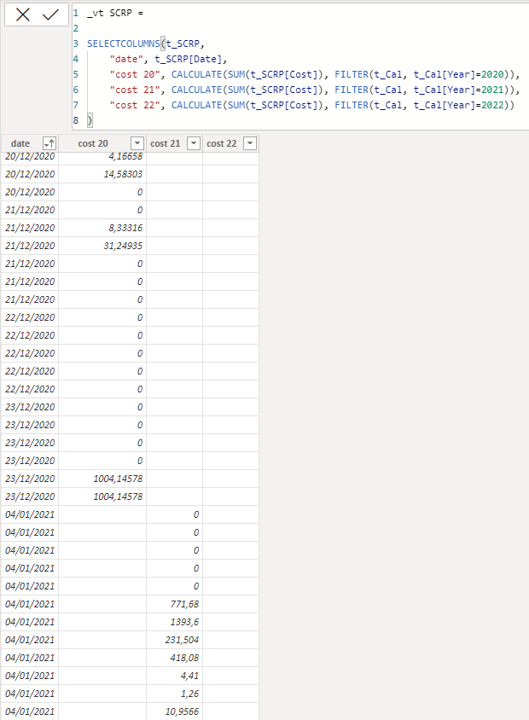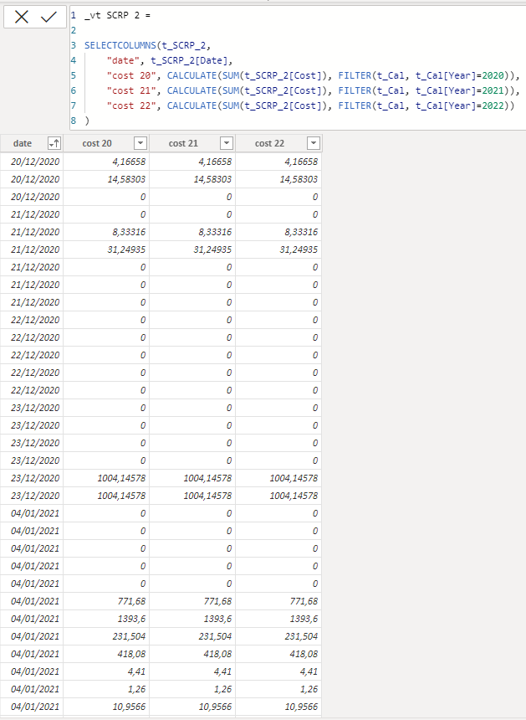cancel
Showing results for
Did you mean:Anonymous
Not applicable

## Same Formula applied on identical Tables gives different outcome

Hi everyone,

I need the valuable help of this fantastic community! I hope someone can explain me the reason why the same formula (SELECTCOLUMNS) applied to two IDENTICAL tables produces very different outcomes!

Picture 'desired outcome' shows what I want to achieve.

It is very important to me have this point clarified because I need SELECTCOLUMNS behaving as per example 1 that is:

- populate 'cost 20' column with all the records of t_SCRP[Cost] filtered by year 2020

- populate 'cost 21' column with all the records of t_SCRP[Cost] filtered by year 2021

- populate 'cost 22' column with all the records of t_SCRP[Cost] filtered by year 2022

- and so on, for upcoming years...

_vt SCRP 1 =
SELECTCOLUMNS(t_SCRP,
"date", t_SCRP[Date],
"cost 20", CALCULATE(SUM(t_SCRP[Cost]), FILTER(t_Cal, t_Cal[Year]=2020)),
"cost 21", CALCULATE(SUM(t_SCRP[Cost]), FILTER(t_Cal, t_Cal[Year]=2021)),
"cost 22", CALCULATE(SUM(t_SCRP[Cost]), FILTER(t_Cal, t_Cal[Year]=2022))
)

_vt SCRP 2 =
SELECTCOLUMNS(t_SCRP_2,
"date", t_SCRP_2[Date],
"cost 20", CALCULATE(SUM(t_SCRP_2[Cost]), FILTER(t_Cal, t_Cal[Year]=2020)),
"cost 21", CALCULATE(SUM(t_SCRP_2[Cost]), FILTER(t_Cal, t_Cal[Year]=2021)),
"cost 22", CALCULATE(SUM(t_SCRP_2[Cost]), FILTER(t_Cal, t_Cal[Year]=2022))
)desired outcomeweird behaviour

Thanks and best regards!

2 ACCEPTED SOLUTIONSSuper User

Can you provide a sample pbix please?Anonymous
Not applicable

Thanks HotChilli, you are right, definitely the problem is into relationships!

Looking more in deep in my file, I also found that other tables giving same 'issue' have a 1:1 relationship with date table while others with *:1 relationship work well. Changing them to many-to-one (and cross filter direction set to 'single') has fixed the issue.

4 REPLIES 4Super User

Yes i got the file, thanks.

The problem is one of the source tables has a relationship with the date table and one does not.  I was going to take a guess that it was relationship based since getting all the same values for a measure usually indicates this.Anonymous
Not applicable

Thanks HotChilli, you are right, definitely the problem is into relationships!

Looking more in deep in my file, I also found that other tables giving same 'issue' have a 1:1 relationship with date table while others with *:1 relationship work well. Changing them to many-to-one (and cross filter direction set to 'single') has fixed the issue.Super User

Can you provide a sample pbix please?Anonymous
Not applicable